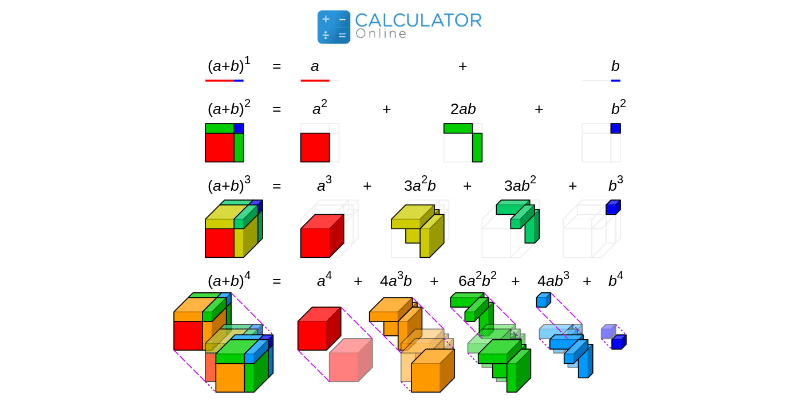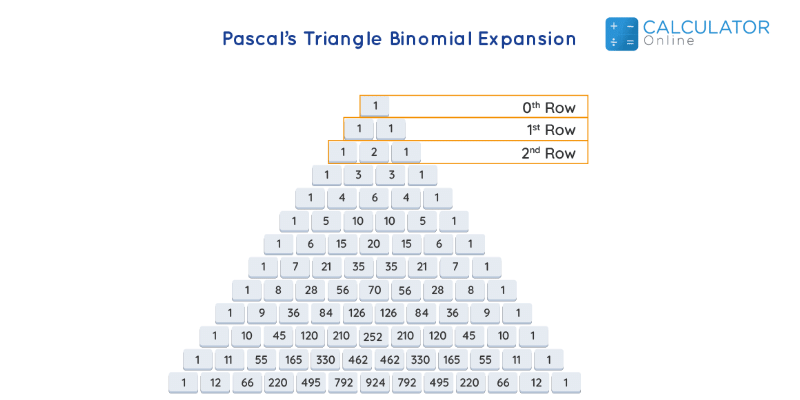•Sign In
• Hire UsUh Oh! It seems you’re using an Ad blocker!

We always struggled to serve you with the best online calculations, thus, there's a humble request to either disable the AD blocker or go with premium plans to use the AD-Free version for calculators.

Or# Binomial Coefficient Calculator

$$\binom{n}{k} = \dfrac{n!}{k!(n-k)!}$$

n:

k:

Table of Content

 1 What is priceeight Class? 2 priceeight Class Chart: 3 How to Calculate priceeight Density (Step by Step): 4 Factors that Determine priceeight Classification: 5 What is the purpose of priceeight Class? 6 Are mentioned priceeight Classes verified by the officials? 7 Are priceeight Classes of UPS and FedEx same?

Get the Widget!

Add this calculator to your site and lets users to perform easy calculations.

Feedback

How easy was it to use our calculator? Did you face any problem, tell us!

The Binomial Coefficient Calculator helps to find the binomial coefficient C(n,k) of two given natural numbers “n” and “k”.

In algebra, a polynomial having two terms is called the binomial, these two terms can be separated by the plus or minus symbols.

## Definition of binomial coefficient:

“The binomial coefficient  C (n, k) is the number of ways of picking “k” unordered possibilities or outcomes from the” possibilities”.

These possibilities are also known as the combination regardless of their symmetry. The binomial coefficient calculator provides us with information about all the possible combinations.The binomial coefficients are used to find the number of possible ways the combination can write the expression.It counts various ways of grouping an unordered collection of items (k) from a total set of items (n), and we can find all the possible combinations by the binomial theorem expansion calculator.

### The binomial coefficient formula:

The binomial coefficient formula is as follows:

$$\binom{n}{k} = \dfrac{n!}{k!(n-k)!}$$### Example:

Consider, we have n=3, and k=2, then the number of all the possibilities or the combination can be calculated as follows:

Solution:

n = 3, k = 2

$$\dbinom{3}{2} = \dfrac{3!}{2!(3-2)!}$$

$$\dbinom{3}{2} = \dfrac{3!}{2!(1)!}$$

$$\dbinom{3}{2} = \dfrac{6}{2(1)}$$

$$\dbinom{3}{2} = \dfrac{6}{2}$$

$$\dbinom{3}{2} = 3$$

Binomial coefficient=3

### Factorials and the Binomial Coefficient:

It is impossible to understand the binomial coefficient concept,without learning about factorial.

The factorial of a natural number “n” is donated by the “n!” and the product of all the natural numbers less than or equal to “n”.

Consider, we want to write  the “7 !”, then we can write it as follows:

7  ! = 7 * 6 * 5 * 4 * 3 * 2 * 1

7 ! = 5040

It can be difficult to find the factorial of larger terms like 49! or 37!, but we can utilize the factorial calculator for the larger terms. The numerical coefficient calculator finds all the combination values normally in a matter of seconds.

### Pascal’s Triangle and Binomial Coefficient:

The Pascal triangle is a simple way to find the coefficient of the binomial expansion. You can evaluate the given binomial coefficient by the pascal triangle in a matter of seconds. Pascal’s triangle is a handy way to  readily verify if the binomial expansion of the given polynomial is done correctly or not.

The generalized formula for the Pascal’s Triangle to find the binomial coefficient are as follows:

(x+y)n = a0 xn y0+ a1 xn-1 y1 + a2 xn-2 y2 + … + an x0 ynYou can find the solution of every binomial coefficient in the Pascal triangle. You can construct any binomial coefficient formula by Pascal’s triangle.

### Example:

Consider the expansion below:

(x+y)2 = x2 + 2xy + y2.

If we are going to write all the hidden terms and the coefficients of this expansion, then we can write:

x2 + 2xy + y2 = 1×2 y0+ 2x1y1 + 1x0y2.

When we see the coefficients, then these are 1,2,1, then we can find this binomial expansion in the second row of Pascal’s triangle. This exact match of the coefficients of the terms in the expansion of (x+y)2. Now if we take the binomial expansion of the polynomial (x+y)n, Then we have the following expression.

Find the binomial coefficient by Pascal’s triangle, which can be used to find the combination by the binomial expansion coefficient calculator. Calculate binomial coefficients and find all the possible combinations by the binomial coefficient. Determine the constant that should be added to the binomial calculator to find all the possible combinations.

### Newton’s Binomial Theory and Binomial Coefficient:

The Newton’s binomial Theory is used to calculate the coefficient of the polynomial raised to the power “n” in a most simple way. We can find the number of possible combination directly by the Newton’s binomial Theory

### Example:

Find the coefficient of the x^4y^2 in the expansion (x+y)^6.

$$\text {coef} (x^4y^2) =\dbinom {6} {4} =\dfrac {6!} {4! \, 2!} = 15$$

We can find the coefficient rather than expanding (x+y)^6, as it can be tedious for us,but you can find the binomial theorem coefficient calculator to find all the possible terms.

The same way, we can find the coefficient of the following terms by Newton’s binomial coefficient theory.

$$\text {coef} (x^6) =\dbinom {6} {6} =\dfrac {6!} {6! \, 0!} = 1$$

$$\text {coef} (x^5y) =\dbinom {6} {5} =\dfrac {6!} {5! \, 1!} = 6$$

$$\text {coef} (x^4y^2) =\dbinom {6} {4} =\dfrac {6!} {4! \, 2!} = 15$$

We have already calculated the values of  x^4y^2 above.

$$\text {coef} (x^3y^3) =\dbinom {6} {3} =\dfrac {6!} {3! \, 3!} = 20$$

$$\text {coef} (x^3y^3) =\dbinom {6} {3} =\dfrac {6!} {3! \, 3!} = 20$$

$$\text {coef} (x^2y^4) =\dbinom {6} {2} =\dfrac {6!} {2! \, 4!} = 15$$

$$\text {coef} (xy^5) =\dbinom {6} {1} =\dfrac {6!} {1! \, 5!} = 6$$

$$\text {coef} (y^6) =\dbinom {6} {0} =\dfrac {6!} {0! \, 6!} = 1$$

There the (x+y)^6, can be deducted by the binomial coefficient calculator:

$$(x+y)^6=x^6+6x^5y+15x^4y^2+20x^3y^3+15x^2y^4+6xy^5+y^6$$

The binomial coefficient formula is simply implemented by Newton’s binomial theory, and we don’t need to implement the whole binomial formula on the algebraic function.

### Working of Binomial Coefficient Calculator:

The binomial factor calculator requires simple input values:

Input:

• Enter the values of “n”
• Enter the value of “k”
• Hit the calculate button to find a binomial coefficient

Output:

The binomial coefficient calculator generates the following result:

• The binomial coefficient
• All the steps are displayed

### What is the binomial probability?

The binomial probability describes the probability of getting the exact probable  result. For example, what is the probability of 2 heads in 3 coin tosses? Find the coefficient a of the term in the expansion by the binomial theorem expansion calculator and  binomial expression and find the probable result.

### What is a binomial expression?

The binomial expression is an algebraic term having two terms, these terms can be separated by the addition or subtraction signs.Evaluate binomial coefficient and find all the possible combinations of the algebraic  terms.The numerical coefficient calculator assists to find all the possible combinations.

### What is the binomial expansion formula?

The formula to find the expansion of a binomial is (a + b)^n = ∑nk=0 (n k) an – k bk, where (n k) = n!/[(n – k)! k!] and n! = 1 . 2 . 3 … n.The binomial-theorem-calculator should be used to find the binomial expansion of any algebraic function. Expanding binomial calculator makes it easy to expand any binomial function.

### When to use the binomial coefficient?

The binomial coefficient is primarily used to count the probability calculations. The main purpose to evaluate binomial coefficients is to find the maximum number of the combination of “k” elements out of “n”. The expanding binomial calculator can be used to find all the possible combinations, in the following example, there are 1906884 combinations possible for given values of “k” elements out of “n”.Use the binomial solver for finding solutions of larger binomial terms.
$$\dbinom{49}{5} =1906884}$$

### Conclusion:

The binomial coefficient is much more than a simple formula, it provides you with all the possible combinations of the “k” elements that can be chosen from a set of “n” elements. The binomial coefficient calculator can implement the binomial coefficient formula in the most efficient way. For finding simplicity, you can use Pascal’s triangle and Newton’s theory to find all the possible combinations.

### References:

From the source of statisticshowto.com:Binomial Coefficient,the importance of the Binomial Coefficient in Statistics

From the source of whitman.edu:Binomial Coefficient, Examples